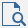Multi Routing - MapleSim Help

Multi Routing

Multiple Heat RoutingDescription The Multi Routing component models multiple Heat Routing between both vectorized ports which routing is controlled by the vectorized integer signal input. The following image is an example with Thermal Conductor components to explain the behavior of Multi Routing component. In the case of $\mathrm{Nodes}$=2, =4, and=[2,3] is as followings.The valid index of the vectorized port is specified by the vectorized input integer signal $\mathrm{sel}$. Heat conductance $\mathrm{G__off}$ in the invalid route is activated. Heat resistance $\mathrm{R__on}$ in the valid route is activated.Equations (For details, see Heat Routing help).Variables

 Symbol Units Description Modelica ID $\mathrm{Q__flow}\left[i\right]$ $W$ Heat flow rate from each port a to each port b The total number of i is determined by numNode*numRoute Q_flow[i] $\mathrm{act_flg}\left[i\right]$ $-$ Active flag The total number of i is determined by numNode*numRoute act_flg[i] $\mathrm{dT}\left[i\right]$ $K$ Relative temperature between each port a and each port b The total number of i is determined by numNode*numRoute dT[i]Connections

 Name Description Modelica ID $\mathrm{port_a}\left[i\right]$ i-th thermal port, a port_a[i] $\mathrm{port_b}\left[i\right]$ i-th thermal port, b port_b[i] $\mathrm{sel}\left[i\right]$ i-th input integer signal to control the condition of contact sel[i]Parameters

 Symbol Default Units Description Modelica ID $\mathrm{Nodes}$ $2$ $-$ Number of nodes of port a numNode $2$ $-$ Number of nodes of port b numRoute $\mathrm{R__on}$ $1.0\cdot {10}^{-7}$ $\frac{K}{W}$ Heat resistance when the each routing is valid. Ron $\mathrm{G__off}$ $1.0\cdot {10}^{-7}$ $\frac{W}{K}$ Heat conductance when the each routing is invalid. GoffSee Also